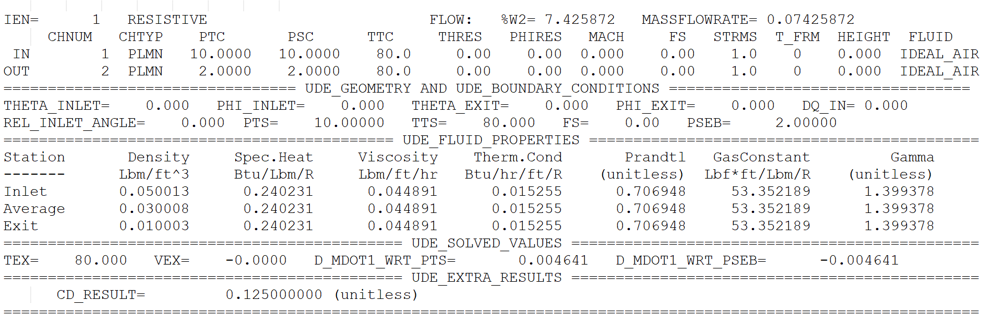# Tutorial 17: User Defined Element

Purpose/Objective

This exercise will walk the user through building a simple flow model using UDE to represent a restriction in the flow.

The user will learn how to:

• Create UDE element with its own Properties
• Implement Python/Fortran Code for the flow equations
• Edit chamber properties
• Check the model
• Run the model
• Post-process the model
• Chamber Types: Plenum
• Element Types: UDE
• Fluid: Air

Step 1: Building Model

1. Open UDE Designer window from Tools → UDE Designer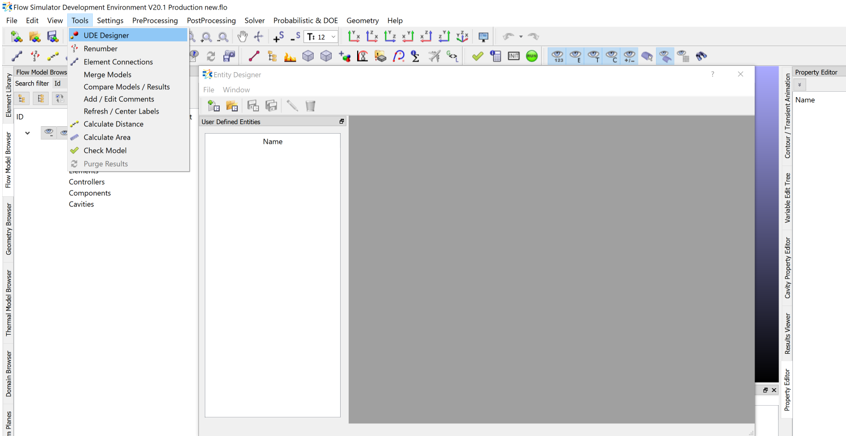2. Create New UDE File by clicking on New (if there is predefined UDE you can also open it from Open)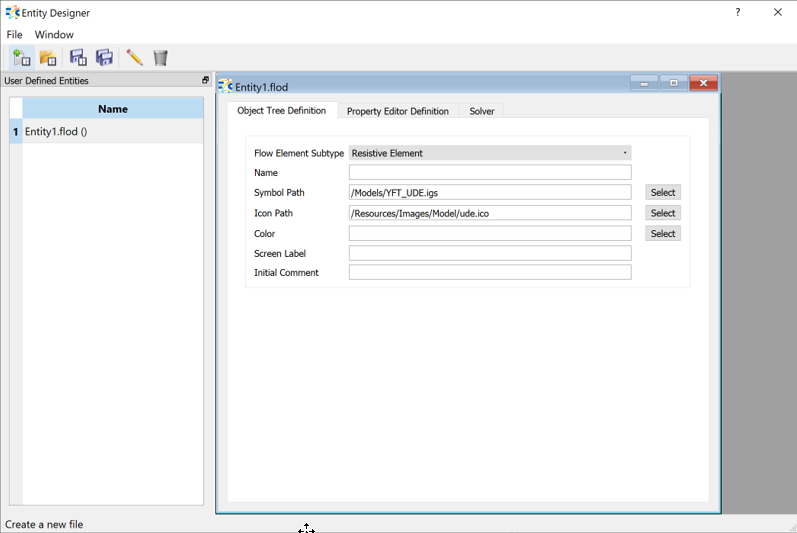3. Select Element Subtype as Resistive Element
4. Give a name (OrificeLikeUDE)
5. Select Symbol Path “/Models/YFT_UDE.igs”
6. Select Icon Path “/Resources/Images/Model/ude.ico”
7. Select color to distinguish UDE element from other elements
8. Save .flod file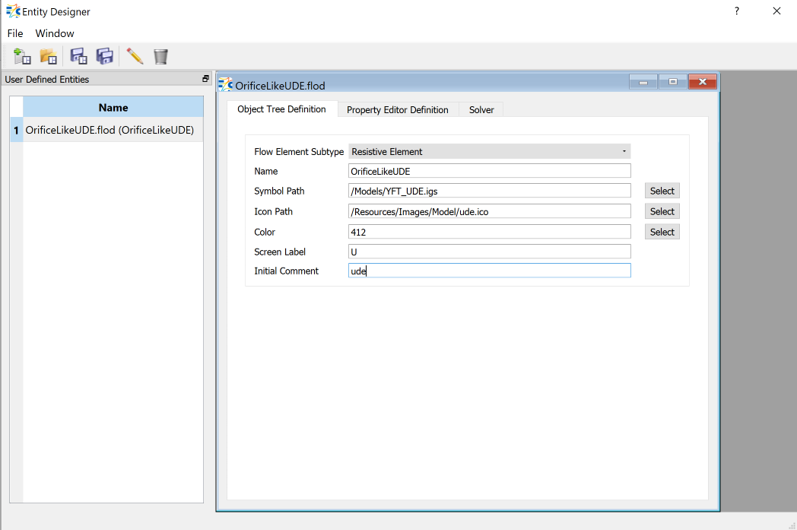9. Add Properties of the UDE element under Property Editor Definition tab
1. DIAMETER: Dia as TEXTBOX with Default value of 2 and Unit Type of Diameter and SAVE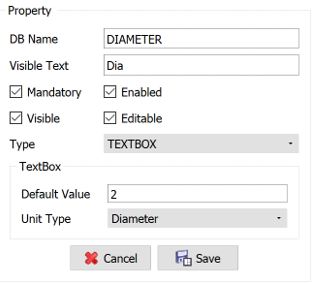2. CD vs Pressure Ratio: Cd_PressureRatio as TABULAR with Default size of 5 and Direction Vertical. Click + to define headers for Pressure Ratio and Cd and then again SAVE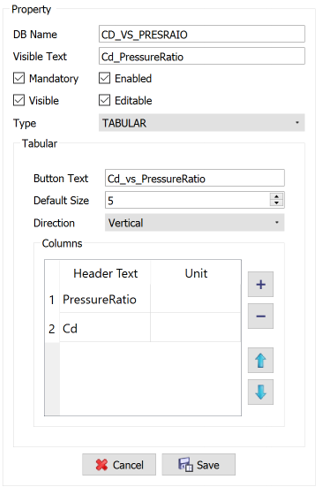3. For Python Based UDE → Select Solver Language as Python & Write Flow Function and Error Traps
# Get user defined properties
DIA = ude.get_scalar_property('DIAMETER')
# Get required properties
PTS = ude.get_boundary_condition(ude.PTS) # upstream pressure (psia)
PSEB = ude.get_boundary_condition(ude.PSEB) # downstream static pressure (psia)
RHO_INLET = ude.get_fluid_property(ude.RHO_INLET) # upstream density (lbm/ft^3)
MU_INLET = ude.get_fluid_property(ude.MU_INLET) # upstream viscosity (lbm/hr/ft)
RHO_EXIT = ude.get_fluid_property(ude.RHO_EXIT) # downstream density (lbm/ft^3)
MU_EXIT = ude.get_fluid_property(ude.MU_EXIT) # downstream viscosity (lbm/hr/ft)
# Get automatically determined flow direction (based on inlet and exit pressures)
#flow_direction = ude.get_flow_direction()
gc = 32.17405
AREA = 0.25 * math.pi * DIA**2 # in^2
print('area=',AREA,flush=True)
PR = PSEB/PTS
print('Pressure Ratio=',PR,flush=True)
N = int(ude.get_array_property('PRESSURERATIO', ude.UDE_ARRAY_SIZE) + 1e-6)
print('number=', N, flush=True)
for I in range(2,N+1):
PR_PREV = ude.get_array_property('PRESSURERATIO', I-1)
PR_NEXT = ude.get_array_property('PRESSURERATIO', I)
CD_PREV = ude.get_array_property('CD', I-1)
CD_NEXT = ude.get_array_property('CD', I)
if ((PR_PREV <= PR) & (PR <= PR_NEXT)):
U = (PR - PR_PREV) / (PR_NEXT - PR_PREV)
CD_calc = (1.0 - U) * CD_PREV + U * CD_NEXT
break
elif ((I == 1) & (PR <= PR_PREV)):
CD_calc = CD_PREV
break
elif (PR_NEXT < 0.001):
CD_calc = CD_PREV
break
elif (I == N):
CD_calc = CD_NEXT
break
if (CD_calc < 0.001):
CD_calc = 0.1
print('cd_calculated=', CD_calc,flush=True)
# Incompressible liquid
mdot = AREA * CD_calc * math.sqrt(2.0 * gc * RHO_EXIT * (PTS - PSEB) / 144.0)
ude.set_solved_value(ude.MDOT1, mdot)
ude.set_extra_result('CD_RESULT' , CD_calc, '(unitless)')
4. For Fortran Based UDE → Select Solver Language as Fortran and follow the steps given there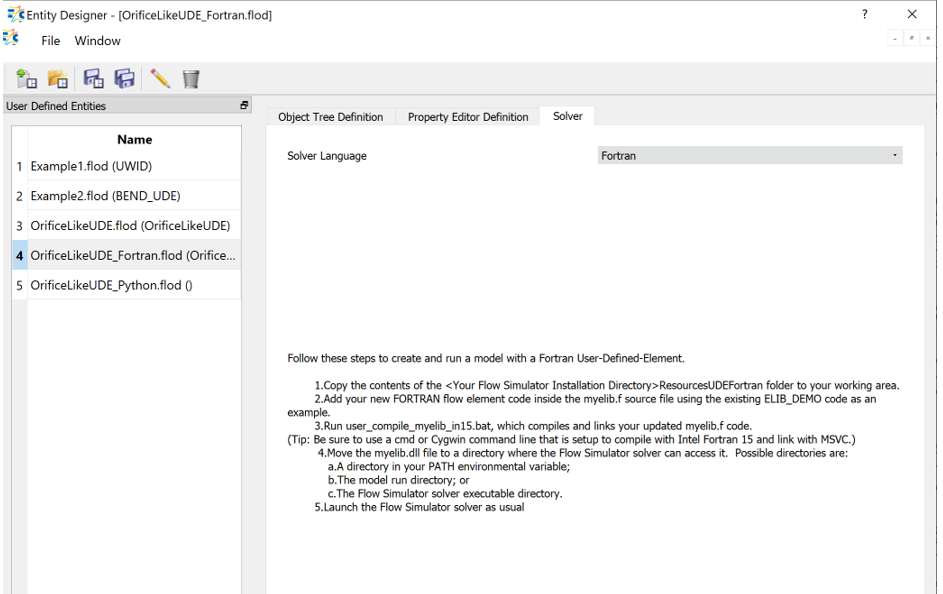5. Update myelib.f code in UDE_SOLVER subroutine
 DIA = GET_UDE_SCALAR_PROPERTY (UDE_ELNUM, 'DIAMETER' , ISTATUS)
! Get required properties
! Retrieve the element-aligned source total pressure (PTS), which is updated every iteration
PTS = GET_UDE_BOUNDARY_CONDITION(UDE_ELNUM, UDE_PTS , ISTATUS)
! Retrieve the element source total temperature (TTS), which is updated every iteration
TTS = GET_UDE_BOUNDARY_CONDITION(UDE_ELNUM, UDE_TTS , ISTATUS)
! Retrieve the element sink static pressure (PSEB), which is updated every iteration
PSEB = GET_UDE_BOUNDARY_CONDITION(UDE_ELNUM, UDE_PSEB , ISTATUS)
! Retrieve the fluid density at element exit (RHO_EXIT), which is updated every iteration, along with other properties
RHO = GET_UDE_FLUID_PROPERTY (UDE_ELNUM, UDE_RHO_EXIT, ISTATUS)
AREA = 0.25 * 3.14D0 * DIA**2 ! in^2
PR = PSEB/PTS
N = N = GET_UDE_ARRAY_PROPERTY(UDE_ELNUM, 'PRESSURERATIO', UDE_ARRAY_SIZE, ISTATUS)
DO I = 2, N
PR_PREV = GET_UDE_ARRAY_PROPERTY(UDE_ELNUM, 'PRESSURERATIO', I-1, ISTATUS)
PR_NEXT = GET_UDE_ARRAY_PROPERTY(UDE_ELNUM, 'PRESSURERATIO', I , ISTATUS)
CD_PREV = GET_UDE_ARRAY_PROPERTY(UDE_ELNUM, 'CD', I-1, ISTATUS)
CD_NEXT = GET_UDE_ARRAY_PROPERTY(UDE_ELNUM, 'CD', I , ISTATUS)
IF (PR_PREV <= PR .AND. PR <= PR_NEXT) THEN
U = (PR - PR_PREV) / (PR_NEXT - PR_PREV)
CD_calc = (1.0D0 - U) * CD_PREV + U * CD_NEXT
EXIT
ELSEIF (I == 1 .AND. PR <= PR_PREV) THEN
CD_calc = CD_PREV ! If PR is off the low end of the lookup-table, use the leftmost CD value
EXIT
ELSEIF (PR_NEXT < 1D-3) THEN
CD_calc = CD_PREV ! If PR is off the high end of the lookup-table, then use the rightmost CD value
EXIT
ELSEIF (I == N) THEN
CD_calc = CD_NEXT ! If PR is off the high end of the lookup-table, then use the rightmost CD value
EXIT
ENDIF
ENDDO
MDOT = CD_calc * (AREA / 144.0D0) * RHO * DSQRT(2.0D0 * 12.0D0 * GC * (PTS - PSEB) / RHO)
! Set flow rate for one stream (MDOT1) so it will be returned to the main solver. This is required.
CALL SET_UDE_SOLVED_VALUE(UDE_ELNUM, UDE_MDOT1 , MDOT , ISTATUS)
! Set TT_EXIT equal to TTS, which is adiabatic. In fact, adiabatic is the default, so you don't really need this line.
CALL SET_UDE_SOLVED_VALUE(UDE_ELNUM, UDE_TT_EXIT , TTS , ISTATUS)
! SET_UDE_EXTRA_RESULT submits a variable that is not needed by the solver to the results print-out so you can see it
CALL SET_UDE_EXTRA_RESULT(UDE_ELNUM, 'CD_RESULT', CD_calc, '(unitless)', ISTATUS) 
1. Upstream Plenum Chamber: Ps = 10 psi, Ts = 80F
2. Downstream Plenum Chamber: Ps = 2 psi, Ts = 80F
7. Drag and Drop OrificeLikeUDE between the Plenum chambers and connect
1. Diameter = 2 in
2. Pressure Ratio vs CD Table as
 PRESSURERATIO CD 0.1 0.1 0.5 0.2 1.0 0.3 2.5 0.4 5 0.5

Step 2: Check Model and Run

1. Select check mark icon from the top toolbar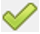to check the model for warnings/errors.
2. Select run icon from toolbar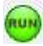. Run Flow Simulator.
3. Outputs of the “print()” functions can be seen on the Run Window

Step 3: Post-process

1. Results file (*.res) should be generated in model running directory.
2. open the result file (*.res) and search for UDE element. Results for UDE will have following data